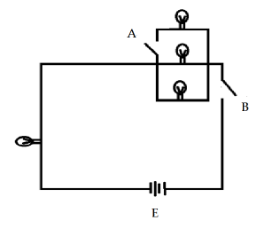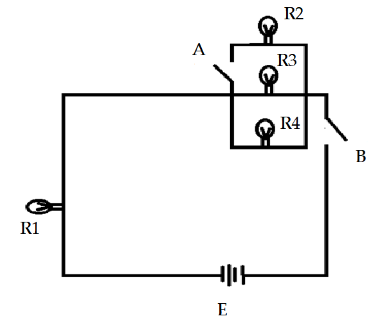# Study the circuit diagram.How many bulbs will light up when switches $A$ and $B$ are closed in different situations?(A) Only switch $A$ is closed(B) Only Switch $B$ is closed, respectively.(A) 4,2(B) 4,3(C) 0,3(D) 0,2Verified
183.3k+ views
Hint: This question can be solved by using the concept of electrical circuits. An electric circuit is a closed path for the circulation of electric current. It consists of a conducting wire, battery, keys, and some electronic devices such as bulbs which are connected in the series.The electric circuit consists of the two switches $A$ and $B$ , and it consists of bulbs $R_1$ , $R_2$ , $R_3$ , and $R_4$ in which $R_2$ , $R_3$ , and $R_4$ are connected in parallel connection while $R_1$ is connected in series with the battery $E$ .
On analysis, it can be seen that when the switch $A$ is closed then the no bulbs will glow because the circuit does not get completed as the switch $B$ is still open. Hence the circuit is incomplete for the current to flow.
While if we close only the switch $B$ and keep the switch $A$ open then the circuit becomes complete. It provides a complete path to the electric current and hence the bulb $R_1$ , $R_3$ and $R_4$ starts glowing but the bulb $R_2$ will not glow as the switch $A$ until not closed.
-If the only switch $A$ is closed then no bulb will light up as the circuit is incomplete.
-If the only switch $B$ is closed then the bulb $R_1$ , $R_3$ and $R_4$ will light up as the circuits are completed but the bulb $R_2$ will not light up as the switch $A$ is not closed.
Here to solve this type of question the concept of the electrical circuits and different types of arrangements of the electronic devices should be known. In this question as to the bulb $R_2$ , $R_3$ , and $R_4$ are connected while bulb $R_1$ and $R_3$ are in series connection.